### Differentiation

In this tutorial, you will learn the following under differentiation through worked examples and interactive applications:

• The concept of gradient of a straight line
• The need of tangents to find the gradient of a curve
• The challenge of drawing an accurate tangent to a curve The issue faced that arises when too many tangents are drawn to a curve
• How differentiation helps us find the gradient of a curve without tangents very accurately
• How to find the differential coefficient from first principles
• Coming up with a formula to find the differential coefficient of a function
• Increasing and decreasing functions
• Stationary points - local maximum, local minimum and point of inflexion
• Modelling with differentiation
• Using differentiation to solve real life problems

The process of finding the gradient or slope of a function is the differentiation.
We used to find the gradient of a straight line, just by dividing the change in 'y' by change in 'x', in a certain range of values. When it comes to a curve, however, it is not easy to find the gradient or slope as the very thing we want to measure, keeps changing from point to point.
Please click the play button at the bottom of the following applet to see it.

we have to draw tangents at all those points and then find the gradients individually. The above animation illustrates just that - and the challenge that we face with.

If we stick to this method we will have to draw hundreds, if not thousands, of tangents to find the gradient at various points of the curve; enough work to put off someone doing maths for years, if not decades, to come!

Good news is that there is a method that comes to our rescue. It is differentiation. It provides us with a method that finds the rate of change of graph; the value so obtained is called differential coefficient (dy/dx) - what we usually call the gradient.

#### Differential Coefficient - dy/dx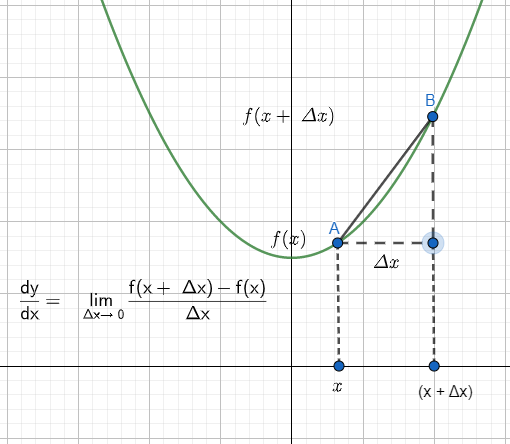In order to find the gradient at a point on a curve, we take into account two points that are very close to each other - A and B. Then the gradient of the line, AB, is calculated while minimizing the distance, AB - or Δx. The gradient, in this circumstances, approaches a limit. It's called the differential coefficient of the function (dy/dx) - or the gradient - at point A.

E.g.1
f(x) = x
f(x + Δx) = x + Δx
f(x + Δx) - f(x) = Δx
[f(x + Δx) - f(x)]/Δx = 1
LimitΔx => 0 [f(x + Δx) - f(x)]/Δx = 1
dy/dx = 1 = 1x0

E.g.2
f(x) = x2
f(x + Δx) = (x + Δx)2
f(x + Δx) - f(x) = (x + Δx)2 - x2
= x2 + 2xΔx + Δx2 - x2
= 2xΔx + Δx2
[f(x + Δx) - f(x)]/Δx = 2x + Δx
LimitΔx => 0 [f(x + Δx) - f(x)]/Δx = 2x
dy/dx = 2x = 2x1

E.g.3
f(x) = x3
f(x + Δx) = (x + Δx)3
f(x + Δx) - f(x) = (x + Δx)3 - x3
= x3 + 3x2Δx + 3xΔx2 + Δx3 - x3
= 3x2Δx + 3xΔx2 + Δx3
[f(x + Δx) - f(x)]/Δx = 3x2 + 3xΔx + Δx2
LimitΔx => 0 [f(x + Δx) - f(x)]/Δx = 3x2
dy/dx = 3x2

The above results - and those of the similar with the use of binominal expansion - lead to the following pattern:

ydy/dx
y = x1dy/dx = 1x0
y = x2dy/dx = 2x1
y = x3dy/dx = 3x2
y = x4dy/dx = 4x3
......
y = xndy/dx = nxn-1

If y = xn then dy/dx = nxn-1

E.g.

Find the differential coefficient of y = 1/x, from the first principles.
y = 1/x
y + Δy = 1/(x + Δx)
So, Δy = 1/(x + Δx) - 1/x
= (x - x - Δx)/x(x + Δx)
= -Δx/x(x + Δx)
Δy/Δx = -1/x(x + Δx)
= -1/(x2 + xΔx)
LimitΔx => 0 Δy/Δx = - 1/x2
dy/dx = - 1/x2

The gradient of a curve at a point is the same as the differential coefficient at the same point; if the curve is in the form of y = xn , its gradient at any point is given by nxn-1 . All we have to do is, to substitute 'x' values for the dy/dx and to get the gradient at points.

E.g.1

Differentiate the following:

1. y = (x + 3)2
y = x2 + 6x + 9
dy/dx = 2x + 6
2. y = x3 - 2x
dy/dx = 3x2 - 2
3. y = x1/2 + 4x
dy/dx = 1/2x-1/2 + 4

E.g.2

y = x2 - 2x

So, dy/dx = 2x -2

Type in the value of x into the textbox and press Enter. The tangent will be drawn at that point

Example

dy/dx =2x -2
dy/dx at x = 0 => dy/dx = 2x0 -2 = -2
dy/dx at x = 1 => dy/dx = 2x1-2 = 0
dy/dx at x = 2 => dy/dx = 2x2 -2 = 2

Just look at the gradients of the tangents; the values from the differentiation are compatible with them.

Using the above curve, y = x2 -2x, find the equations of tangents at x = 0 and x = 2. The two tangents meet up at A. Find the coordinates of A as well.

dy/dx = 2x - 2
dy/dx at x =0 => dy/dx = -2
For the tangent,
x = 0; y = 0; m = dy/dx = -2
y = mx + c
0 = -2x0 + c => c = 0
So, the equation of the tangent is y = -2x.
dy/dx at x = 2 => dy/dx = 2
For the tangent,
x = 2; y = 0; m = dy/dx = 2
y = mx + c
0 = 2x2 + c => c = -4
So, the equation of the tangent is y = 2x - 4.
If two equations cross at A, -2x = 2x - 4
4x = 4
x = 1
y = -2 x 1 = -2
So, the coordinates of A are, (1,-2).

E.g.1

The equation of a curve is y = x² -x -6. Find the gradient of the curve at x = -2 and x = 3. Find the coordinates of the point where the gradient is zero.
y = x² -x -6
dy/dx = 2x - 1
When x = -2
dy/dx = 2 x -2 - 1 = -5
When x = 3
dy/dx = 2 x 3 - 1 = 5
When the gradient is zero, dy/dx = 0
2x - 1 = 0
x = 0.5
Sub into y = x² -x -6,
y = 0.5² - 0.5 - 6 = - 6.25
The coordinates are, (0.5, -6.25)

E.g.2
The equation of a curve is y = 4x - x². Find the gradient of the curve at x = 1. Find the coordinates of the point where the gradient is -4. y = 4x - x²
dy/dx = 4 - 2x
When x = 1
dy/dx =4 - 2 x 1 = 2
When the gradient is -4, dy/dx = -4
4 - 2x = -4
2x = 8
x = 4
Sub into y = 4x - x²
y = 4 x 4 - 16 = 0
The coordinates are, (4, 0)

E.g.3

The equation of a curve is y = x³ - 4x. Find the coordinates of the point/s where the gradient is 8. Comment on the answer.
y = x³ - 4x
dy/dx = 3x² - 4
When the gradient is 8, dy/dx = 8
3x² - 4 = 8
3x² = 12
x² = 4
x = ± 2
There are two points on the curve with the same gradient, x = 2 and x = -2
Sub into y = x³ - 4x
The coordinates of the two points are, (-2, 0) and (2,0).
The following graph shows how it happens:#### Equations of tangents and normal to a curveSince we know how to find the gradient of a curve at any point, we are now in a position to extend the knowledge to find the equation of a tangent and a normal - perpendicular line - easily, because both are just straight lines.

Example

The equation of a curve is y = x² -2x. Sketch the curve and find the equations of the tangent and that of the normal at x = 3.
y = x² - 2x
dy/dx = 2x - 2
At x = 3,
dy/dx = 4
y = x² -2x = 9- 6 = 3
The coordinates of the point are (3, 6)
For the tangent,
y - 3 = 4 (x - 3)
y - 3 = 4x - 12
y = 4x - 9
For the normal, the gradient = -1/4.
y - 3 = -1/4 (x - 3)
4y - 12 = -x + 3
4y + x = 15
The following applet shows both the tangent and the corresponding normal at x = 3.

Practice

Find the equations of both the tangent and the normal at the following points of the above curve. Then drag the point A on the grid and check your answers. The equations of both lines are updated automatically. x = 0
x = -1
x = 2

Example

Find the equations of the tangent and the normal to the curve, y = x²/4, at the point P, where x = 4. The normal crosses the curve again at Q. Find the coordinates of the point Q.
At P,
x = 4; y = 4²/4 = 4; (4,4)
dy/dx = x/2
dy/dx = m = 4/2 = 2
For the tangent,
y - 4 = 2(x - 4)
y = 2x - 8 + 4
y = 2x - 4
For the normal,
m = -1/2; x = 4; y = 4
y - 4 = -1/2 (x-4)
2y - 8 = -x + 4
y = (-x + 12)/2
When the curve and the normal intersect again,
x²/4 = (-x + 12)/2
x²/2 = -x + 12
x² = -2x + 24
x² + 2x - 24 = 0
(x + 6)(x - 4) = 0
x = -6 or x = 4
The coordinates of Q ~ (-6, 9).
This is the curve that shows the tangent and normal at P as well as point Q.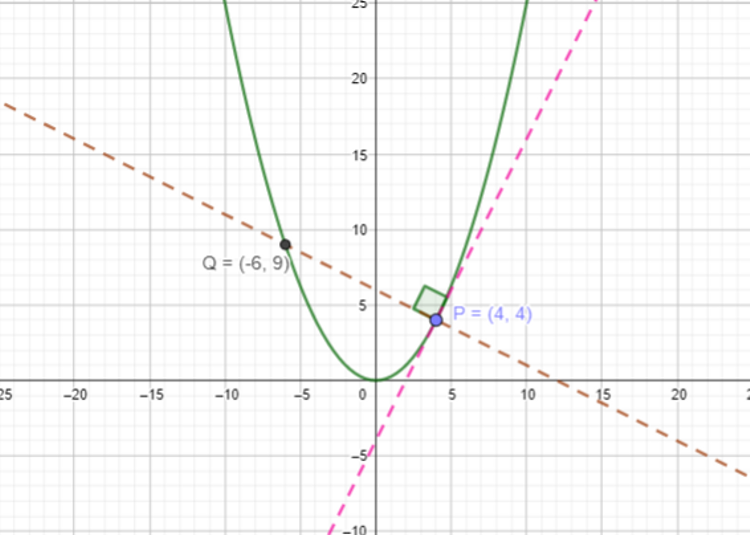### Increasing and Decreasing Functions

Increasing function: If the differential coefficient of a function is positive, dy/dx > 0, in a certain range of x, it is said to be an increasing function in that range.

If dy/dx > 0 or f'(x) > 0 => then f(x) is an increasing function.

Decreasing function: If the differential coefficient of a function is positive, dy/dx < 0, in a certain range of x, it is said to be an decreasing function in that range.

If dy/dx < 0 or f'(x) < 0 => then f(x) is a decreasing function.

To illustrate this, you can use the following applet. Just slide the point, P, along the curve and see how it can become an increasing or a decreasing function, depending on its position.

### Stationary Points or Turning Points

A point on a curve with zero gradient is called a stationary point or a turning point on the curve. There are three types of stationary points.

• Local maximum
• Local minimum
• A point of inflection

They are as follows:Local Maximum

• dy/dx = 0
• d²y/dx² < 0

Example

Find the position of the stationary point on the curve, y = 2x - x², its nature and the coordinates.
y = 2x - x²
dy/dx = 2 - 2x
At the stationary point, dy/dx = 0
2 - 2x = 0
x = 1
Now, in order to determine its nature, let's find the second derivative.
d²y/dx² = -2
d²y/dx² < 0
It's a local maximum; the curve is shown below.
x = 1; y = 2x1 - 1² = 1
Coordinates of the stationary point are, (1, 1)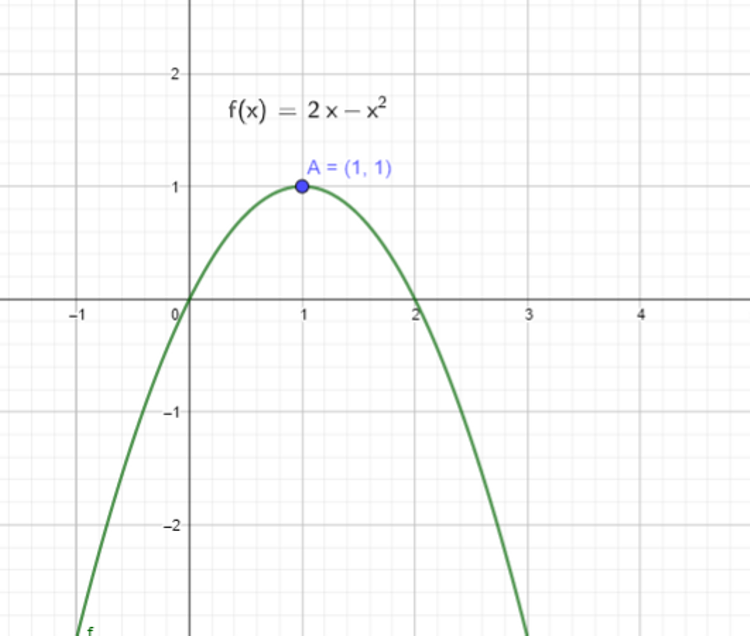Local Minimum

• dy/dx = 0
• d²y/dx² > 0

Example

Find the position of the stationary point on the curve, y = x² - 2x - 3, its nature and the coordinates.
y = x² - 2x - 3
dy/dx = 2x - 2
At the stationary point, dy/dx = 0
2x - 2x = 0
x = 1
Now, in order to determine its nature, let's find the second derivative.
d²y/dx² = 2
d²y/dx² > 0
It's a local minimum; the curve is shown below.
x = 1; y = 1² - 2 - 3 = -4
Coordinates of the stationary point are, (1, -4)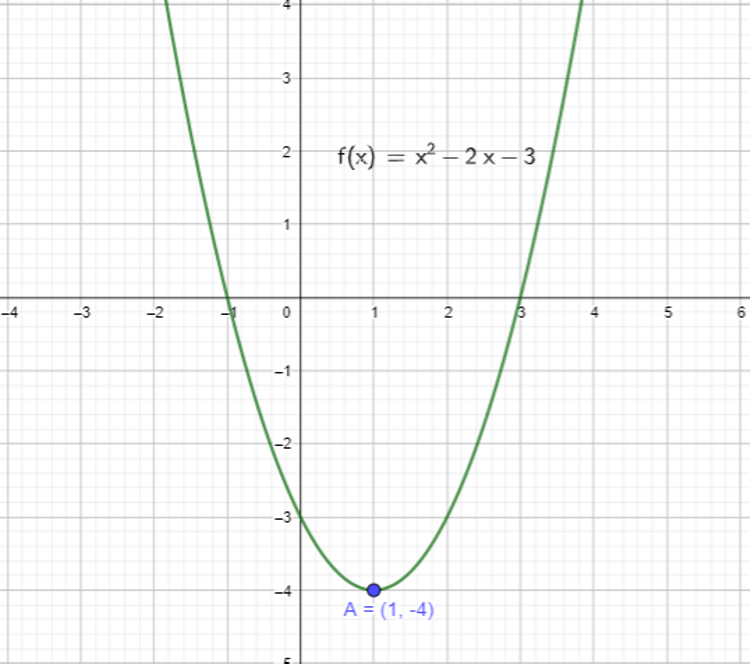Point of Inflection

• dy/dx = 0
• d2y/dx2 = 0
• d3y/dx3 ≠ 0

E.g. 1

The equation of a curve is y = x3 - 2. Find the stationary point on it and its nature.
y = x3 - 2
dy/dx = 3x2
At the stationary point, dy/dx = 0
3x2 = 0
x = 0 d2y/dx2 = 6x
At x = 0,
d2y/dx2 = 6(0) = 0
d3y/dx3 = 6
d3y/dx3 ≠ 0
The stationary point is a point of inflection.

The following diagram shows the point of inflection.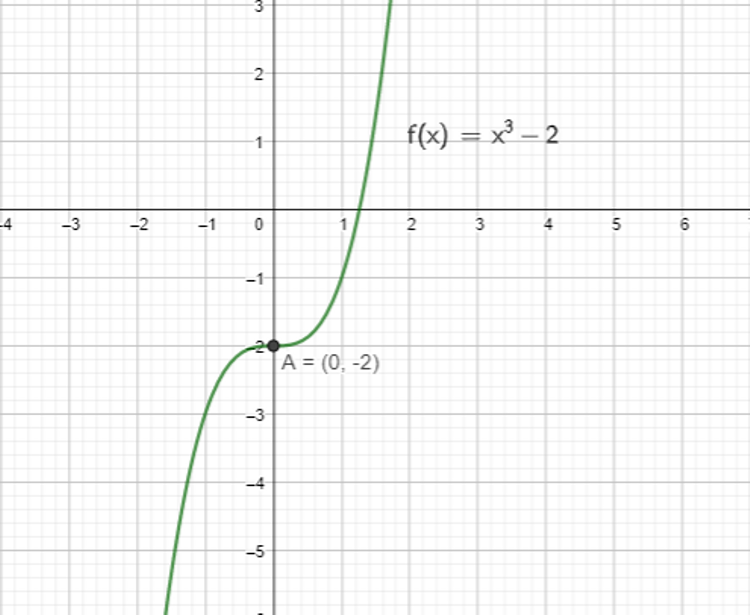Example 2

Check whether there is a stationary point on the curve, y = x4. y = x4
dy/dx = 4x3
At the stationary point, if any, dy/dx = 0
4x3 = 0
x = 0
d²y/dx² = 12x2
At x = 0,
d²y/dx² = (12)02 = 0
d3y/dx3 = 24x
At x = 0
d3y/dx3 = (24)0 = 0
It's not a point of inflection; nor is it a stationary or turning point. it's just a turning region.

The following diagram explains it.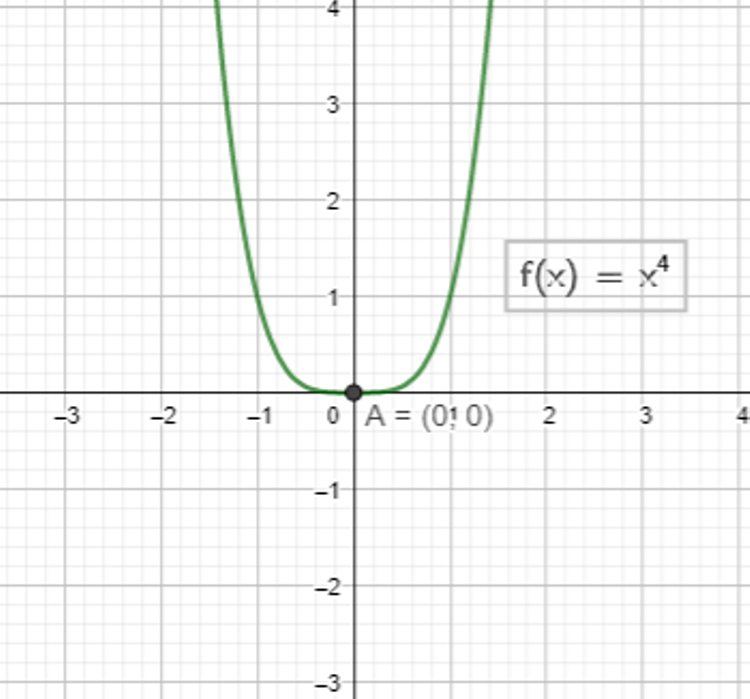E.g. 3

Find the number of the stationary points on the curve, y = x3- x2 - 2x, their nature and the coordinates.
y = x3- x2 - 2x
dy/dx = 3x2 -2x - 2
At the stationary points, dy/dx = 0
3x2 -2x - 2 = 0
x = (-(-2) ± √(-2)² - (4)(3)
(-2))/(2(3))
x = -0.55 or x = 1.22 d²y/dx² = 6x - 2
At x = -0.55
d2y/dx2 = 6(-0.55) - 2 = -5.3 < 0
The stationary point at x = -0.55 is a maximum.
At x = -0.55, y = y = -0.553- (-0.55)2 - 2(-0.55) = 0.63
The coordinates are, (-0.55, 0.63)
At x = 1.22
d2y/dx2 = 6(1.22) - 2 = 5.3 > 0
The stationary point at x = 1.22 is a minimum.
At x = 1.22, y = y = 1.223- (1.22)2 - 2(1.22) = -2.11
The coordinates are, (1.22, -2.11)

The following diagram shows the stationary points.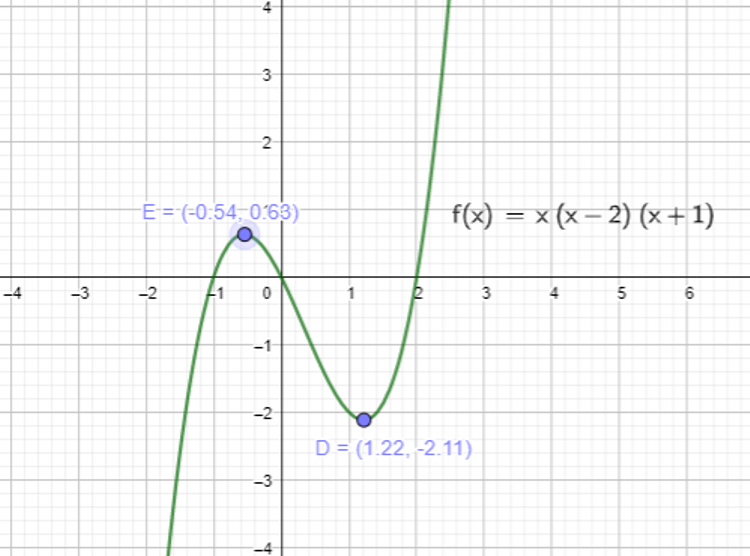#### Additional tests to determine the type of stationary point

In addition to go by the second derivative - or even the third in the case of point of inflection - there are other tests that you can carry out in order to find the nature of a turning point:

• You can check the value of the function, before and after the stationary point
• You can check the gradient of the function, before and after the stationary point

The following table shows the conditions:

Stationary Point f(x) - before f(x) - after dy/dx - before dy/dx - after
Maximum Smaller Smaller Positive Negative
Minimum Bigger Bigger Negative Positive
Point of Inflection Smaller or Bigger Bigger or Smaller Positive / Positive Negative or Negative

### Modelling with Differentiation

Differentiation is widely used in the fields of economics, biology and many more, when it comes to optimizing the variables involved. As you go through the relatively simple examples that I present here, you will get a clear idea about its potential in achieving the above goal.

In real life situations, we often turn dy/dx into forms like ds/dt, dv/dt, dA/dt, dl/dt to represent the rate of change of distance, velocity, area and length respectively, when the denominator is linked to time.

E.g.1

A farmer wants to make an enclosure for his sheep using a fencing material of length 60m with one side being a wall. He wants to make its shape rectangular so that he can keep as many sheep as possible. How should he choose the dimensions for the enclosure.
Let x be the width. Then the length is (60 - 2x) - only three sides to cover, as fourth is the wall.
If the area is A,
A = x(60 - 2x)
A = 60x - 2x2

When the area is maximum, the gradient is 0
dA/dx = 60 -4x
dA/dx =0 => 60 - 4x = 0
4x = 60
x = 15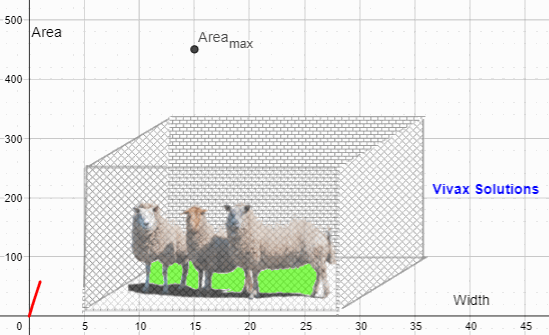So, he should choose the width as 15m and the length as (60 - 2x15) = 30m, to maximize the area - a brilliant use of differentiation!

These are the possibilities - in a spread sheet with the curve:

E.g.2

Four squares from the corners of a square plate are removed so that it can be turned into a open cubical box. Find the length of a square to be removed in terms of the length of the main square so that the volume of the box is a maximum.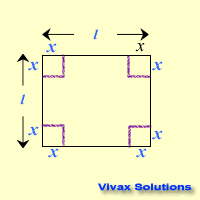Let the length of the plate be l and that of a small square be x.
If the volume is V,
V = x(l - 2x)2
= x(l2 - 4lx + 4x2)
= l2x - 4lx2 + 4x3
dV/dx = l2 - 8lx + 12x2
At the peak of the graph of V against x, dV/dx = 0
12x2 - 8lx + l2 = 0
x = 8l ± √ 64l2 - 48l2 / 24
x = 8l ± √ 16l2 / 24
x = 8l ± 4l / 24
x = l/2 or l/6
Since l/2 is not possible, x = l/6

Therefore, to maximize the volume, a square of side l/6 should be cut off from each corner of the plate.
If the length is 12 cm, the model is as follows: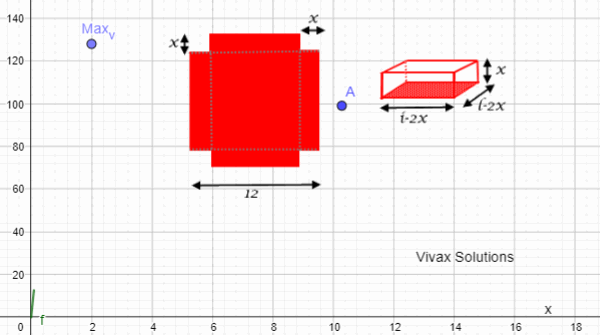E.g.3

The product of two positive numbers is 100. Find the numbers so that the sum of them is a minimum.
Let the numbers be x and 100/x
If the sum is S, then S = x + 100/x
So, dS/dx = 1 - 100/x2
If the sum is a minimum, dS/dx = 0
1 - 100/x2 = 0
x2 = 100
x = 10.
So, the numbers are 10 and 10.
Let's verify the result:

100, 1101
50, 252
25, 429
20, 525
10, 1020 ☺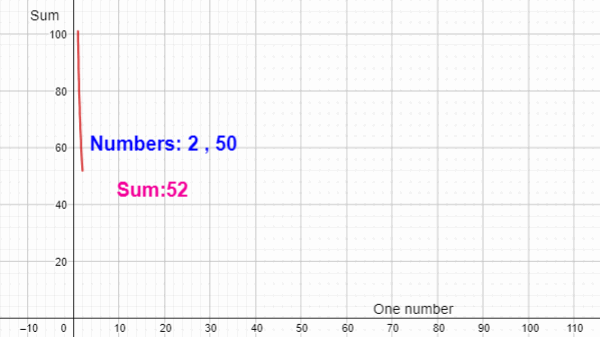E.g. 4

The radius of a circular oil drop is expanding at 3 mm/s, when the radius is 8 mm. Find the rate of change of circumference and area.
C = 2πr; A = πr²; dr/dt = 3; r = 3
dC/dr = 2π; dA/dr = 2πr
dC/dt = dC/dr X dr/dt = 2π X 3 = 6π mm/s
dA/dt = dA/dr X dr/dt = 2πr X dr/dt = 6π X 3 = 18π mm²/s

E.g. 5

The radius of a spherical bubble is expanding at 2 mm/s, when the radius is 6 mm. Find the rate of change of surface area and volume.
A = 4πr²; V = 4/3 πr³; dr/dt = 2; r = 6
dA/dr = 8πr; dV/dr = 4πr²
dA/dt = dA/dr X dr/dt = 8π X 6 X 2 = 96π mm²/s
dV/dt = dV/dr X dr/dt = 4πr² X dr/dt = 4π X 36 X 2 = 288π mm³/s

#### Questions for Practice

Please work out the following questions to complement what you have just learnt.

1. A container has a mixture of 125cm³, cement. It has to be turned into a block of square cross section in such a way that its surface area is a minimum. Find the required length.
2. The height of a ball thrown up against time can be modelled by the equation, y = 16t - 4t². Calculate the maximum height reached by the ball.
3. The area of a paper is 72cm². The margins at top and bottom are 3cm and on the sides 2cm. What are the dimensions, if the printed area is a maximum ?
4. A small-scale farmer estimates that if he plucks his strawberries during the Covid-19 lockdown, now, he will have 120 kg, worth £1 per kg, but if he waits, the crop will grow 20 kg per week, while the price will drop 10p per kg per week. When should he pluck them to get the largest cash returns?
5. The perimeter of a sector is 16 cm. Find the radius of the sector, if the area is to be a maximum.
6. A local air-condition company finds there is a net profit of £15 per unit in summer, if the production can be kept below 1000 units. If they make over 1000 units in proportion to the demand, the profit per unit decreases 1p for each unit above 1000. How many units altogether would give the maximum net profit?
7. A solid rectangular column has a square base. The sum of its height and a side of the base is 12 m. If the density of the material is 8000 kg/m³, find the maximum mass of the column.
8. The width of a rectangular block is one half of its length. Find its length, when the volume is a maximum and total surface area is 108 cm².
9. The sum of the the length and the circumference of the base of a cylinder is 10 m. Find the maximum volume of the cylinder.
10. Show that the surface area of a cylinder is maximum, when its diameter is equal to the height.

Move the mouse over, just below this, to see the answers:

1. 5
2. 16
3. 10.4, 6.9
4. 2
5. 4
6. 1250
7. 2048000
8. 6
9. 11.8Maths is challenging; so is finding the right book. K A Stroud, in this book, cleverly managed to make all the major topics crystal clear with plenty of examples; popularity of the book speak for itself - 7th edition in print.

### Recommended - GCSE & iGCSEThis is the best book available for the new GCSE(9-1) specification and iGCSE: there are plenty of worked examples; a really good collection of problems for practising; every single topic is adequately covered; the topics are organized in a logical order.

### Recommended for A LevelThis is the best book that can be recommended for the new A Level - Edexcel board: it covers every single topic in detail;lots of worked examples; ample problems for practising; beautifully and clearly presented.